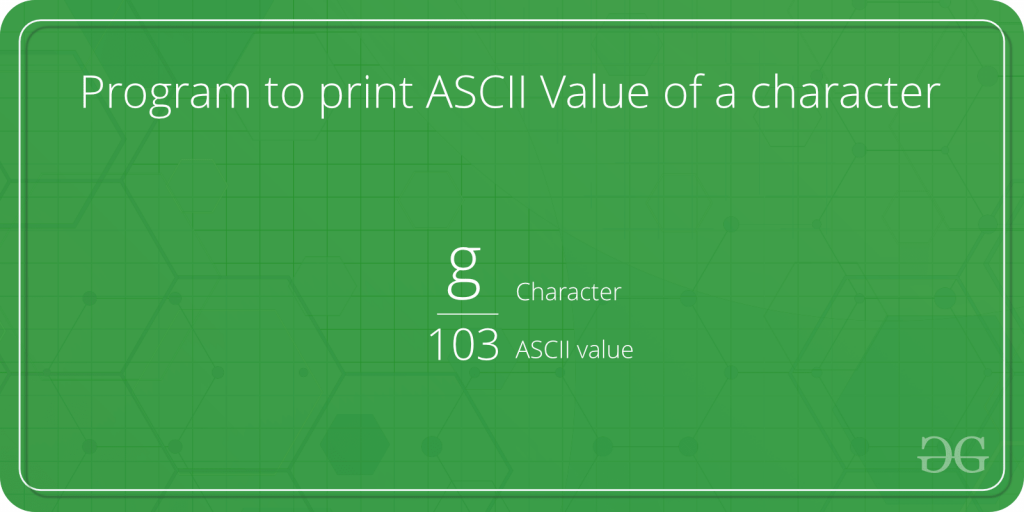# Program to print ASCII Value of a character

Given a character, we need to print its ASCII value in C/C++/Java/Python.Examples :

```Input : a
Output : 97

Input : D
Output : 68
```

Here are few methods in different programming languages to print ASCII value of a given character :

1. Python code using ord function :
ord() : It coverts the given string of length one, return an integer representing the unicode code point of the character. For example, ord(‘a’) returns the integer 97.

 `# Python program to print  ` `# ASCII Value of Character ` ` `  `# In c we can assign different ` `# characters of which we want ASCII value  ` ` `  `c ``=` `'g'` `# print the ASCII value of assigned character in c ` `print``(``"The ASCII value of '"` `+` `c ``+` `"' is"``, ``ord``(c)) `

Output:

```The ASCII value of g is 103
```
2. C code: We use format specifier here to give numeric value of character. Here %d is used to convert character to its ASCII value.

 `// C program to print ` `// ASCII Value of Character ` `#include ` `int` `main() ` `{ ` `    ``char` `c = ``'k'``; ` ` `  `    ``// %d displays the integer value of a character ` `    ``// %c displays the actual character ` `    ``printf``(``"The ASCII value of %c is %d"``, c, c); ` `    ``return` `0; ` `} `

Output:

```The ASCII value of k is 107
```
3. C++ code: Here int() is used to convert character to its ASCII value.

 `// CPP program to print ` `// ASCII Value of Character ` `#include ` `using` `namespace` `std; ` `int` `main() ` `{ ` `    ``char` `c = ``'A'``; ` `    ``cout << ``"The ASCII value of "` `<< c << ``" is "` `<< ``int``(c); ` `    ``return` `0; ` `} `

Output:

```The ASCII value of A is 65
```
4. Java code : Here, to find the ASCII value of c, we just assign c to an int variable ascii. Internally, Java converts the character value to an ASCII value.

 `// Java program to print ` `// ASCII Value of Character ` `public` `class` `AsciiValue { ` ` `  `    ``public` `static` `void` `main(String[] args) ` `    ``{ ` ` `  `        ``char` `c = ``'e'``; ` `        ``int` `ascii = c; ` `        ``System.out.println(``"The ASCII value of "` `+ c + ``" is: "` `+ ascii); ` `    ``} ` `} `

5. C# code : Here, to find the ASCII value of c, we just assign c to an int variable ascii. Internally, C# converts the character value to an ASCII value.

 `// C# program to print ` `// ASCII Value of Character ` `using` `System; ` ` `  `public` `class` `AsciiValue  ` `{ ` `    ``public` `static` `void` `Main() ` `    ``{ ` ` `  `        ``char` `c = ``'e'``; ` `        ``int` `ascii = c; ` `        ``Console.Write(``"The ASCII value of "` `+  ` `                        ``c + ``" is: "` `+ ascii); ` `    ``} ` `} ` ` `  `// This code is contributed  ` `// by nitin mittal `

Output:

```The ASCII value of e is 101
```

My Personal Notes arrow_drop_upCheck out this Author's contributed articles.

If you like GeeksforGeeks and would like to contribute, you can also write an article using contribute.geeksforgeeks.org or mail your article to contribute@geeksforgeeks.org. See your article appearing on the GeeksforGeeks main page and help other Geeks.

Please Improve this article if you find anything incorrect by clicking on the "Improve Article" button below.

Improved By : nitin mittal

6

Please write to us at contribute@geeksforgeeks.org to report any issue with the above content.